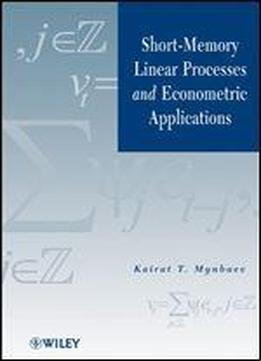# Short-memory Linear Processes And Econometric ApplicationsTags: Kairat T. Mynbaev

This book serves as a comprehensive source of asymptotic results for econometric models with deterministic exogenous regressors. Such regressors include linear (more generally, piece-wise polynomial) trends, seasonally oscillating functions, and slowly varying functions including logarithmic trends, as well as some specifications of spatial matrices in the theory of spatial models. The book begins with central limit theorems (CLTs) for weighted sums of short memory linear processes. This part contains the analysis of certain operators in Lp spaces and their employment in the derivation of CLTs. The applications of CLTs are to the asymptotic distribution of various estimators for several econometric models. Among the models discussed are static linear models with slowly varying regressors, spatial models, time series autoregressions, and two nonlinear models (binary logit model and nonlinear model whose linearization contains slowly varying regressors). The estimation procedures include ordinary and nonlinear least squares, maximum likelihood, and method of moments. Additional topical coverage includes an introduction to operators, probabilities, and linear models Lp-approximable sequences of vectors convergence of linear and quadratic forms regressions with slowly varying regressors spatial models convergence nonlinear models and tools for vector autoregressions.